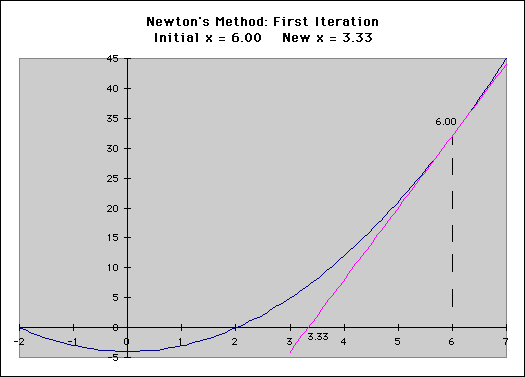## Calculating interest rates using newton’s methodHere’s a post I wrote for Microfinance Transparency on March 5, 2010

In a previous blog post, I described how to use a spreadsheet like Microsoft Excel to calculate interest rates. Again, interest rate calculations are at the core of MFTransparency’s ability to provide accurate data that can be compared across various products offered by numerous MFIs. In the last post we looked at Excel’s IRR and XIRR functions and concluded that XIRR is more accurate because it takes into account the actual payment dates of the loan and thus allows us to calculate annualized interest rates even with irregular repayment schedules.

But for the more technical among us, I realize that even this may not be sufficient. Today I’m going to demonstrate how to write a computer program that is as accurate as Excel 2007’s XIRR function. This article is likely to be of less broad interest, but it provides transparency into how we will calculate interest rates for future data collection trips; and it may be useful for MFIs that wish to automate interest rate calculations for a larger data set than can be handled with Excel.

• EIR = cf*(1+rate)^n

cf = cashflow, n=number of periods/year

### Simple Guess and Check

The first technique is to make a guess about the interest rate and then run the numbers through the EIR formula to see how close you are. You then iterate, guessing somewhere in the middle of your previous guesses, or widening your area by doubling. The advantage of this technique is that it is simple and it gets you the right answer eventually (or at least fairly accurately given enough guesses).

### Newton’s method

A more advanced way to solve the EIR formula is to use Calculus. It’s still a “guessing” technique, but it is much more efficient and elegant.

It’s easier to visualize this technique if we draw a graph and plot an initial guess, with the goal of finding the point on the graph where it crosses the x axis.

We start by making an initial guess and then figuring out what the “tangent” line at that point would be. This is the same thing as the derivative of the EIR calculation:• EIR = cf*(1+rate)^n
• f’rate = cf*n*(rate+1)^(n-1) = pink tangent line is the derivative

cf = cashflow, n=number of periods/year

We can then figure out where the tangent line intersects the x axis and use that to make a much more accurate second guess.

The speed advantage the Newton-Raphson method has over a simple guess and check technique is quite remarkable. It is common to be able to achieve a result that matches Excel to within 8 decimal places in 5-10 iterations.

### Implementing Newton-Raphson

Fortunately for me, I didn’t have to implement the entire Newton-Raphson algorithm myself because the programming language I use already has a library to do this. I just give it the EIR function and the derivative function and it does the rest.

For the programmers out there, here are a few links to implementations in various languages:

• C#: uses bisection rather than Newton’s method
• Java
• Python

### Related Information:

For those of you who would like to know more, please explore the following links: